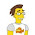## Sunday, March 25, 2012

### Faking it with higher rank (Existential Quantification pt. 2)

In my previous post I provided a short background on Existential Quantification.  In this post, I'll provide an alternative for some uses and suggest why it may be preferred.

Suppose we want to create a data store that uses lists for small amounts of data, but otherwise uses vectors.  We can easily use E.Q.

``````import Data.Vector (Vector)
import qualified Data.Vector as V
class Buffer c where
type El c :: *
init :: Int -> El c -> c
(!)  :: c -> Int -> El c
push :: c -> El c -> c

instance Buffer [a] where
type El [a] = a
init = replicate
(!)  = (!!)
push lst el = el : init lst

instance Buffer (Vector a) where
type El (Vector a) = a
init = V.replicate
(!)  = (V.!)
push vec el = V.cons el \$ V.unsafeInit vec

data EBuffer a = forall c. (Buffer c, El c ~ a) => EBuffer c

initBuffer :: Int -> EBuffer Double
initBuffer sz
| sz < 10   = EBuffer (init sz 0 :: [Double])
| otherwise = EBuffer (init sz 0 :: Vector Double)
``````
and now we have our existentially-quantified buffer.

Unfortunately this code doesn't offer anywhere near the hoped-for performance (at least not for the problem that led to this post).  The problem is that now that the actual type of buffer is hidden in the EBuffer data, the compiler is no longer able to inline function calls to (!) or push.  Instead it needs to go through the dictionary methods, adding a lot of extra overhead.  Even worse, a lot of further optimization opportunities are lost.

It turns out that there is another approach that doesn't suffer from this problem.  The approach is based on higher-rank types.  Normal Haskell code uses rank-1 type variables, which means that any variables in a type signature are instantiated by the calling code.  However, with the RankNTypes extension, it's possible to write code of any rank desired.  Consider the function
``````hr1 :: (forall a. a -> a) -> (Char, Int)
hr1 myId = (myId 'a', myId 0)
``````
The first parameter to hr1 is a rank-2 function.  It needs to be a function that can take an input of any type, and return a value with the same type.  The only total function that can do this is id, and calling hr1 id returns exactly what we would expect.

(What happens if you change the type signature to hr1 :: (a -> a) -> (Char, Int) ? )

Armed with higher-rank types, we can replace the existentially-quantified type in two steps:

1.  Refactor whichever code uses the data so that it's in the form someFunc :: Buffer buf => buf -> AResult.  This part usually isn't too difficult.

2.  Create another function like following:
``````runWithBuffer
:: Int
-> (forall buf. (Buffer buf, El buf ~ Double) => buf -> b)
-> b
runWithBuffer sz fn
| sz < 10   = let buf = init sz 0 :: [Double]
in fn buf
| otherwise = let buf = init sz 0 :: Vector Double
in fn buf

``````
With our new runWithBuffer function, we've successfully decoupled the buffer selection algorithm from the usage site without using existential quantification.  Plus, in many cases GHC generates better code with more opportunities for further optimization.

A slightly more extensive example is available in my dsp-extras codebase.  The class and various buffer types are in the Data.RingBuffer modules.  The buffers are used in Language.AuSL.CompileArr, with the function optimizeBufType performing the duties of runWithBuffer.

1.That inefficiency problem will not exist with a regular non-existentially quantified type, such as:
data Ebuffer a where Ebuffer :: (Buffer a) => EBuffer a
am I correct? Can this data type be used instead to accomplish the same task?

1.Unfortunately, using a GADT in this manner is essentially the same as existential quantification (see http://blog.ezyang.com/2011/03/type-tech-tree/ for more details), and suffers from the same problem. Consider this simplified example:

data GDT a where
GDT :: (Show a, Num a) => a -> GDT a

test2 gdt = case gdt of
GDT n -> show \$ 2*n

compiled at -O2, this results in the core

Foo.test2 :: forall a_a9R. Foo.GDT a_a9R -> GHC.Base.String
[GblId,
Arity=1,
Str=DmdType S,
Unf=Unf{Src=, TopLvl=True, Arity=1, Value=True,
ConLike=True, Cheap=True, Expandable=True,
Guidance=IF_ARGS  110 0}]
Foo.test2 =
\ (@ a_af3) (gdt_aab :: Foo.GDT a_af3) ->
case gdt_aab of _ { Foo.GDT \$dShow_af5 \$dNum_af6 n_aac ->
GHC.Show.show
@ a_af3
\$dShow_af5
(GHC.Num.*
@ a_af3
\$dNum_af6
(GHC.Num.fromInteger @ a_af3 \$dNum_af6 Foo.test3)
n_aac)
}

so it's still going through the dictionary methods.

This is actually the same core that's produced for "data EQu = forall a. (Show a, Num a) => EQu a", modulo naming.